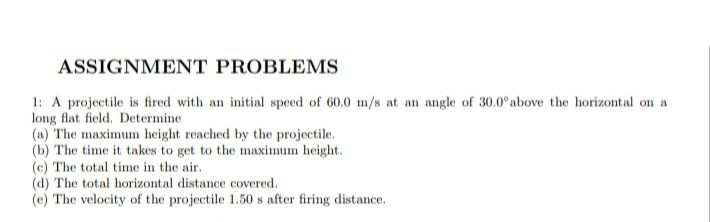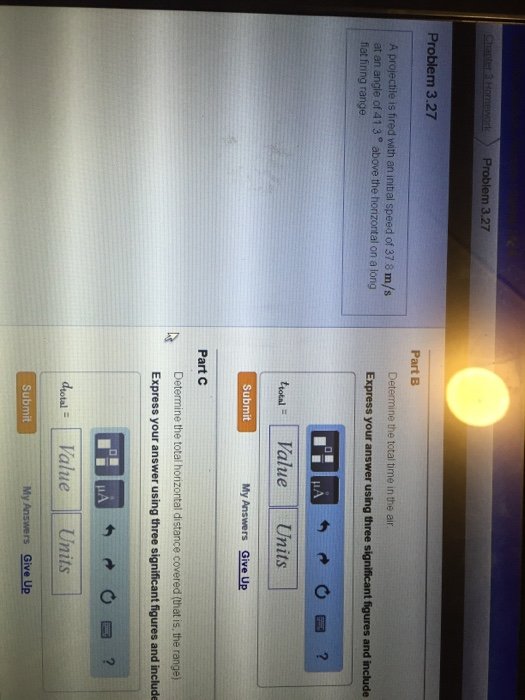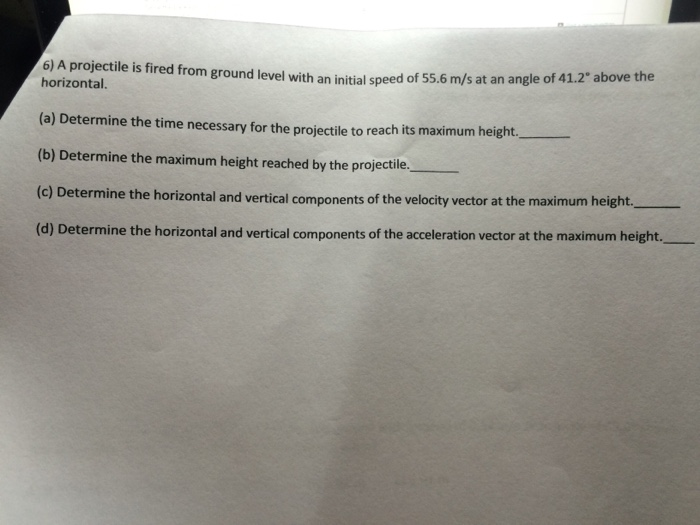Question

# A projectile is fired with an initial speed of 10 m/s. Find theangle of projection...

A projectile is fired with an initial speed of 10 m/s. Find the angle of projection such that the maximum height of the projectile is equal to 0.9 times its horizontal range.

Initial speed = 10 m/s

Let the angle of projection be with the horizontal.

So, vertical component of initial speed = 10 sin

So,

Total K.E. initially = 1/2 m (10)^2  (independent of ?

K.E. in the vertical direction = 1/2 m (10 sin ?)^2

K.E. in the horizontal direction = 1/2 m (10 cos ?)^2

At maximum height, K.E in vertical direction = 0  (since velocity in the vertical direction is 0)

but it gains P.E. by gaining the height h.

So,

Gain of P.E = Loss of K.E.

=> m g h max = 1/2  m (10 sin ?)^2

=> h max = (10 sin ? )^2  / 2g  =  (50/9.8) sin^2 ? = 5.102 sin^2 ?

Time taken for attaining h max =  h max / average speed  = 5.102 sin^2 ? / (10 sin ? + 0)/2 = 1.0204 sin ?

Distance travelled horizontally when height is  h max = 10 cos ? * 1.0204 sin ? = 10.204 sin ? cos ?

Projectile reaches maximum height and comes back down and hits the ground.

So,

Total horizontal distance travelled

= 2 * horizontal distance travelled when at h max

= 2 * 10.204 sin ? cos ?

= 20.408 sin ? cos ?

Given that :

h max = 0.9 total horizontal distance travelled

=> 5.102 sin^2 ? = 0.9 * 20.408 sin ? cos ?

=> sin ? / cos ? = 0.9 * 20.408 / 5.102

=> tan ? = 3.6

=> ? = 74.48 º  (with the horizontal)  (ANSWER).

The Maximum height reached by the projectile is given by

Vy2 =Voy2+2ay*dY

Since final Velocity is zero

0=(10Sin(o))2+2*(-9.8)*dY

dY =(10Sin(o))2/19.6

The time of flight the projectile is twice time needed to reach the maximum height

t=2*(Vy-Voy)/a =2*(0-10sin(o))/(-9.8)

t=-20Sin(o)/9.8

the range of projectile is

dX =Voxt =(10Cos(o))*20Sin(o)/9.8

given

dY =0.9dX

(10Sin(o))2/19.6 =0.9*(10Cos(o))*20Sin(o)/9.8

tan(o) =3.6

o=tan-1(3.6) =74.5o

Initial speed = 10 m/s

Let the angle of projection be with the horizontal.

So, vertical component of initial speed = 10 sin

So,

Total K.E. initially = 1/2 m (10)^2  (independent of ?

K.E. in the vertical direction = 1/2 m (10 sin ?)^2

K.E. in the horizontal direction = 1/2 m (10 cos ?)^2

At maximum height, K.E in vertical direction = 0  (since velocity in the vertical direction is 0)

but it gains P.E. by gaining the height h.

So,

Gain of P.E = Loss of K.E.

=> m g h max = 1/2  m (10 sin ?)^2

=> h max = (10 sin ? )^2  / 2g  =  (50/9.8) sin^2 ? = 5.102 sin^2 ?

Time taken for attaining h max =  h max / average speed  = 5.102 sin^2 ? / (10 sin ? - 0)/2 = 1.0204 sin ?

Distance travelled horizontally when height is  h max = 10 cos ? * 1.0204 sin ? = 10.204 sin ? cos ?

Projectile reaches maximum height and comes back down and hits the ground.

So,

Total horizontal distance travelled

= 2 * horizontal distance travelled when at h max

= 2 * 10.204 sin ? cos ?

= 20.408 sin ? cos ?

Given that :

h max = 0.9 total horizontal distance travelled

=> 5.102 sin^2 ? = 20.408 sin ? cos ?

=> sin ? / cos ? = 20.408 / 5.102

=> tan ? = 4

=> ? = 75.964 º  (with the horizontal)  (ANSWER).

> Please correct the last 4 lines as under :
=> 5.102 sin^2 𝛉 = 0.9 * 20.408 sin 𝛉 cos 𝛉
=> sin 𝛉 / cos 𝛉 = 0.9 * 20.408 / 5.102
=> tan 𝛉 = 3.6
=> 𝛉 = 74.48 º (with the horizontal) (ANSWER).

Tulsiram Garg Tue, Oct 26, 2021 8:29 AM

#### Earn Coins

Coins can be redeemed for fabulous gifts.

Similar Homework Help Questions
• ### A projectile is fired with an initial speed of 20. m/s at an angle of 42.2°...

A projectile is fired with an initial speed of 20. m/s at an angle of 42.2° above the horizontal on a long flat firing range. Determine, a) the maximum height reached by the projectile, b) the total time in the air, c) the total horizontal distance covered (that is, the range), d) the speed of the projectile 1.50 s after firing. e) direction of the speed at 1.50 s.

• ### A projectile is fired with an initial speed of 250 m/s and angle of elevation 60°.

A projectile is fired with an initial speed of 250 m/s and angle of elevation 60°. The projectile is fired from a position 100 m above the ground. (Recall g ≈ 9.8 m/s2. Round your answers to the nearest whole number.) (a) Find the range of the projectile. (b) Find the maximum height reached. (c) Find the speed at impact.

• ### A projectile is fired with an initial speed of 37.4 m/s at an angle of 45.0...

A projectile is fired with an initial speed of 37.4 m/s at an angle of 45.0 ° above the horizontal on a long flat firing range.Part ADetermine the maximum height reached by the projectile. Express your answer using three significant figures and include the appropriate units. Part B Determine the total time in the air Part C Determine the total horizontal distance covered (that is, the range).

• ### A projectile is fired with an initial speed of 250 m/s and angle of elevation 60°

A projectile is fired with an initial speed of 250 m/s and angle of elevation 60°. (Recall g ≈ 9.8 m/s2. Round your answers to the nearest whole number.) (a) Find the range of the projectile. (b) Find the maximum height reached. (c) Find the speed at impact.

• ### ASSIGNMENT PROBLEMS 1: A projectile is fired with an initial speed of 60.0 m/s at an...ASSIGNMENT PROBLEMS 1: A projectile is fired with an initial speed of 60.0 m/s at an angle of 30.0°above the horizontal on a long flat field. Determine (a) The maximum height reached by the projectile. (b) The time it takes to get to the maximum height. (c) The total time in the air. (d) The total horizontal distance covered. (e) The velocity of the projectile 1.50 s after firing distance.

• ### A projectile is fired in such a way that its horizontal range is equal to 9.5...

A projectile is fired in such a way that its horizontal range is equal to 9.5 times its maximum height. What is the angle of projection?

• ### A projectile is fired with an initial speed of 37.8 m/s at an angle of 41...A projectile is fired with an initial speed of 37.8 m/s at an angle of 41 3 degree above the horizontal on a long flat fining range

• ### A projectile is fired with an initial speed of 36.2m/s at an angle of 41.1 degrees...

A projectile is fired with an initial speed of 36.2m/s at an angle of 41.1 degrees above the horizontal on a long flat firing range. determine maxiumum height reached by projectile total time in the air determine the range speed of the projectile 1.80s after firing

• ### With steps please A projectile is fired from ground level with an initial speed of 55.6...With steps please A projectile is fired from ground level with an initial speed of 55.6 m/s at an angle of 41.2 above the horizontal. (a) Determine the time necessary for the projectile to reach its maximum height. (b) Determine the maximum height reached by the projectile. (c) Determine the horizontal and vertical components of the velocity vector at the maximum height. (d) Determine the horizontal and vertical components of the acceleration vector at the maximum height.